#### 2020-04-10

Radiant’s goal is to provide access to the power of R for business analytics and data science. Although Radiant’s web-interface can handle many data and analysis tasks, you may prefer to write your own code. Radiant provides a bridge to programming in R(studio) by exporting the functions used for analysis. For example, you can run your analyses in Radiant and output the relevant function calls to an R or Rmarkdown document. Most pages in the app have an icon on the bottom left of your screen that you can click to generate a (reproducible) report with your analysis in the Report > Rmd (or Report > R) tab. As an alternative to clicking the icon you can also press `ALT-enter` on your keyboard. Click the `Knit report` button on the Report > Rmd page to render the report to HTML or press the `Save report` button to produce a Notebook, HTML, PDF, Word, or Rmarkdown file. Radiant’s function manuals can be viewed using the commands below:

``````help(package = radiant.data)

You can also use Rstudio to render and edit Rmarkdown documents generated in Radiant. When you install and load Radiant it exports functions that can be called from R-code and/or an Rmarkdown document. For example, you can paste the commands below into the command console to get the same output as in the browser interface.

``````library(radiant)
data(diamonds, envir = environment())
result <- single_mean(diamonds, "price")
summary(result)``````
``````Single mean test
Data      : diamonds
Variable  : price
Confidence: 0.95
Null hyp. : the mean of price = 0
Alt. hyp. : the mean of price is not equal to 0

mean     n n_missing        sd     se      me
3,907.186 3,000         0 3,956.915 72.243 141.651

diff     se t.value p.value   df     2.5%    97.5%
3907.186 72.243  54.084  < .001 2999 3765.535 4048.837 ***

Signif. codes:  0 '***' 0.001 '**' 0.01 '*' 0.05 '.' 0.1 ' ' 1``````
``plot(result)``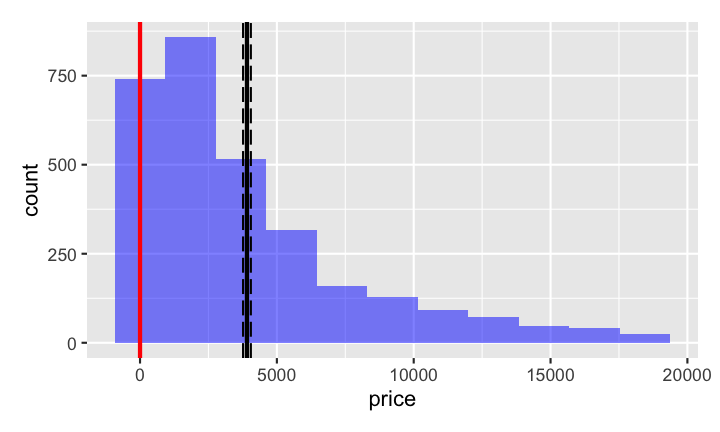You can also call functions for visualization (see below) and access help from the console using `?visualize`

``````visualize(
diamonds,
xvar = "carat",
yvar = "price",
type = "scatter",
facet_row = "clarity",
color = "clarity",
labs = labs(title = "Diamond Prices (\$)"),
custom = FALSE
) ``````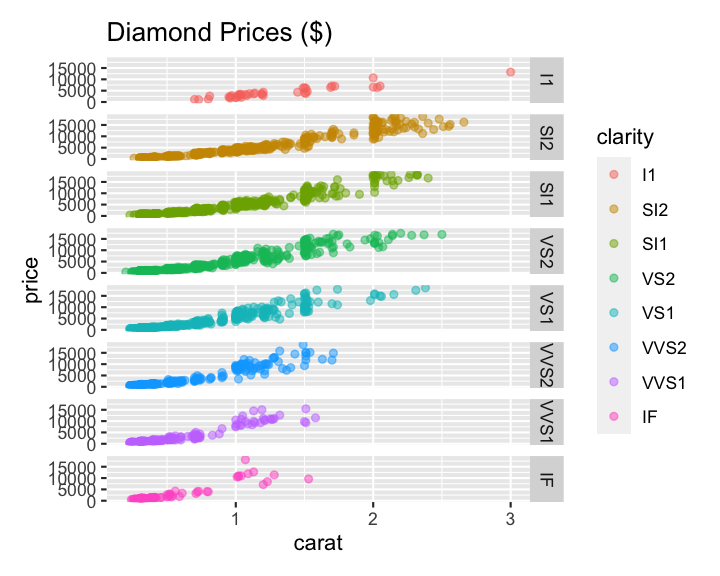Use `library(radiant)` to load the library. To see the index of functions currently available in, for example, Radiant’s Model menu use the `help(package = "radiant.model")` command

Lets start by comparing the mean of a variable to a (population) value using R’s built-in `mtcars` dataset. This functionality is in the Radiant menu Basics > Means > Single mean. The analysis is conducted in function `single_mean`. Calling the `summary` method on the result object will show tabular output. Calling `plot` on the same result object will produce relevant plots.

``````result <- single_mean(
mtcars,
var = "mpg",
comp_value = 20,
alternative = "greater"
)
summary(result)``````
``````Single mean test
Data      : mtcars
Variable  : mpg
Confidence: 0.95
Null hyp. : the mean of mpg = 20
Alt. hyp. : the mean of mpg is > 20

mean  n n_missing    sd    se    me
20.091 32         0 6.027 1.065 2.173

diff    se t.value p.value df     5% 100%
0.091 1.065   0.085   0.466 31 18.284  Inf

Signif. codes:  0 '***' 0.001 '**' 0.01 '*' 0.05 '.' 0.1 ' ' 1``````
``plot(result, plots = "hist")``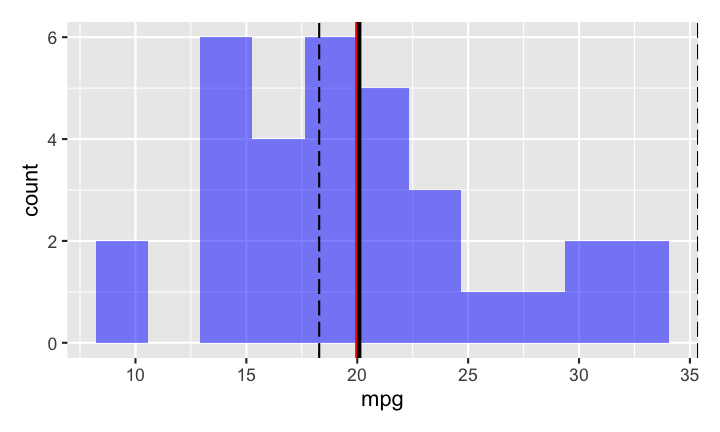To compare the mean price of diamonds across different levels of clarity we can call the `compare_means` function:

``````result <- compare_means(
diamonds,
var1 = "clarity",
var2 = "price",
)
summary(result)``````
``````Pairwise mean comparisons (t-test)
Data      : diamonds
Variables : clarity, price
Samples   : independent
Confidence: 0.95

clarity      mean   n n_missing        sd      se      me
I1 4,194.775  40         0 2,519.678 398.396 805.832
SI2 5,100.189 529         0 4,406.982 191.608 376.407
SI1 3,998.577 721         0 3,813.190 142.011 278.804
VS2 3,822.967 661         0 3,917.484 152.372 299.193
VS1 3,789.181 442         0 3,880.907 184.596 362.797
VVS2 3,337.820 284         0 3,932.706 233.363 459.348
VVS1 2,608.460 224         0 3,201.282 213.895 421.513
IF 2,411.697  99         0 3,330.560 334.734 664.268

Null hyp.     Alt. hyp.                diff     p.value
I1 = SI2      I1 not equal to SI2      -905.414 1
I1 = SI1      I1 not equal to SI1       196.198 1
I1 = VS2      I1 not equal to VS2       371.808 1
I1 = VS1      I1 not equal to VS1       405.594 1
I1 = VVS2     I1 not equal to VVS2      856.955 1
I1 = VVS1     I1 not equal to VVS1     1586.315 0.023   *
I1 = IF       I1 not equal to IF       1783.078 0.025   *
SI2 = SI1     SI2 not equal to SI1     1101.612 < .001  ***
SI2 = VS2     SI2 not equal to VS2     1277.222 < .001  ***
SI2 = VS1     SI2 not equal to VS1     1311.008 < .001  ***
SI2 = VVS2    SI2 not equal to VVS2    1762.369 < .001  ***
SI2 = VVS1    SI2 not equal to VVS1    2491.729 < .001  ***
SI2 = IF      SI2 not equal to IF      2688.492 < .001  ***
SI1 = VS2     SI1 not equal to VS2      175.610 1
SI1 = VS1     SI1 not equal to VS1      209.396 1
SI1 = VVS2    SI1 not equal to VVS2     660.757 0.446
SI1 = VVS1    SI1 not equal to VVS1    1390.117 < .001  ***
SI1 = IF      SI1 not equal to IF      1586.880 0.001   ***
VS2 = VS1     VS2 not equal to VS1       33.786 1
VS2 = VVS2    VS2 not equal to VVS2     485.146 1
VS2 = VVS1    VS2 not equal to VVS1    1214.507 < .001  ***
VS2 = IF      VS2 not equal to IF      1411.270 0.005   **
VS1 = VVS2    VS1 not equal to VVS2     451.361 1
VS1 = VVS1    VS1 not equal to VVS1    1180.721 0.001   ***
VS1 = IF      VS1 not equal to IF      1377.484 0.012   *
VVS2 = VVS1   VVS2 not equal to VVS1    729.361 0.606
VVS2 = IF     VVS2 not equal to IF      926.123 0.68
VVS1 = IF     VVS1 not equal to IF      196.763 1

Signif. codes:  0 '***' 0.001 '**' 0.01 '*' 0.05 '.' 0.1 ' ' 1``````
``plot(result, plots = c("bar", "density"))``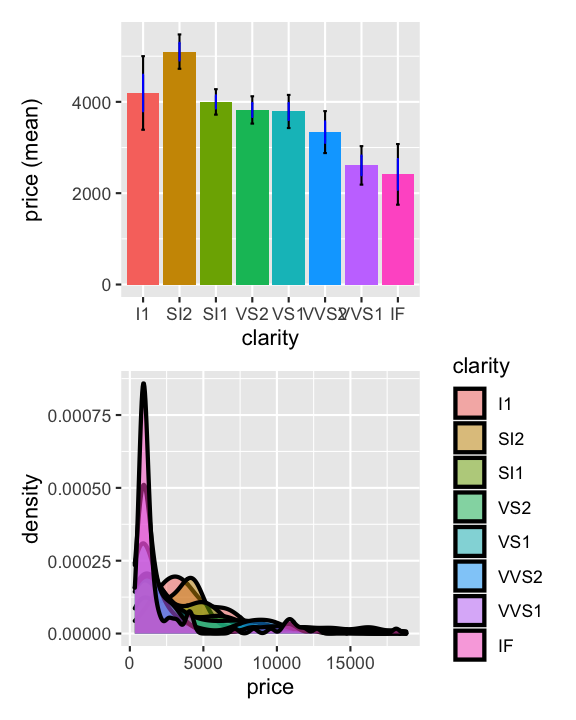These datasets are available after loading the radiant library by using the `data` function. We can also load data through Radiant’s browser interface and then access the data from the console after closing the app. Start radiant using the command below and then click select `Examples` from the `Load data of type` dropdown in the Data > Manage tab. Then close the app by clicking the icon in the navbar and then clicking `Stop`. The datasets loaded through the web-interface are now available in the `r_data` environment as well. To use them directly in your code use `attach(r_data)`.

``````## start radiant in Rstudio, load the example data, then click the power
## icon in the navigation bar and click on Stop

Because we already loaded the radiant library we already have access to all the data we need here. Lets use the `compare_means` function to evaluate salary data for professors of different ranks using:

``````result <- compare_means(salary, var1 = "rank", var2 = "salary")
summary(result)``````
``````Pairwise mean comparisons (t-test)
Data      : salary
Variables : rank, salary
Samples   : independent
Confidence: 0.95

rank        mean   n n_missing         sd        se        me
AsstProf  80,775.985  67         0  8,174.113   998.627 1,993.823
AssocProf  93,876.438  64         0 13,831.700 1,728.962 3,455.056
Prof 126,772.109 266         0 27,718.675 1,699.541 3,346.322

Null hyp.              Alt. hyp.                         diff      p.value
AsstProf = AssocProf   AsstProf not equal to AssocProf   -13100.45 < .001  ***
AsstProf = Prof        AsstProf not equal to Prof        -45996.12 < .001  ***
AssocProf = Prof       AssocProf not equal to Prof       -32895.67 < .001  ***

Signif. codes:  0 '***' 0.001 '**' 0.01 '*' 0.05 '.' 0.1 ' ' 1``````
``plot(result)``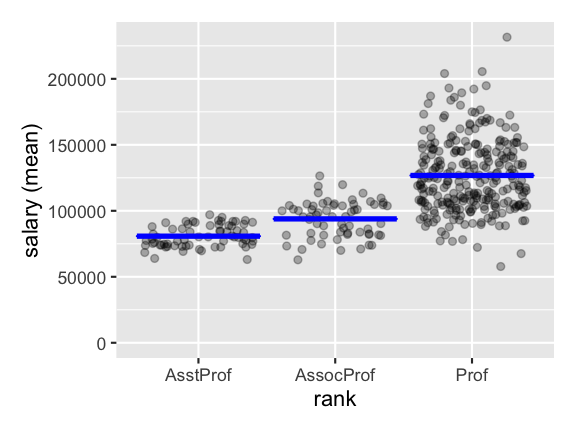We can also run regressions and get output in a format that would require quite a few lines of code to produce from scratch:

``````result <- regress(diamonds, rvar = "price", evar = c("carat","clarity"))
summary(result, sum_check = "confint")``````
``````Linear regression (OLS)
Data     : diamonds
Response variable    : price
Explanatory variables: carat, clarity
Null hyp.: the effect of x on price is zero
Alt. hyp.: the effect of x on price is not zero

coefficient std.error t.value p.value
(Intercept)    -6780.993   204.952 -33.086  < .001 ***
carat           8438.030    51.101 165.125  < .001 ***
clarity|SI2     2790.760   201.395  13.857  < .001 ***
clarity|SI1     3608.531   200.508  17.997  < .001 ***
clarity|VS2     4249.906   201.607  21.080  < .001 ***
clarity|VS1     4461.956   204.592  21.809  < .001 ***
clarity|VVS2    5109.476   210.207  24.307  < .001 ***
clarity|VVS1    5027.669   214.251  23.466  < .001 ***
clarity|IF      5265.170   233.658  22.534  < .001 ***

Signif. codes:  0 '***' 0.001 '**' 0.01 '*' 0.05 '.' 0.1 ' ' 1

F-statistic: 3530.024 df(8,2991), p.value < .001
Nr obs: 3,000

coefficient      2.5%     97.5%     +/-
(Intercept)    -6780.993 -7182.855 -6379.131 401.862
carat           8438.030  8337.834  8538.227 100.196
clarity|SI2     2790.760  2395.873  3185.646 394.886
clarity|SI1     3608.531  3215.384  4001.679 393.148
clarity|VS2     4249.906  3854.604  4645.208 395.302
clarity|VS1     4461.956  4060.801  4863.111 401.155
clarity|VVS2    5109.476  4697.311  5521.640 412.165
clarity|VVS1    5027.669  4607.574  5447.764 420.095
clarity|IF      5265.170  4807.024  5723.317 458.147``````
``````pred <- predict(result, pred_cmd = "carat = 1:10")
print(pred, n = 10)``````
``````Linear regression (OLS)
Data                 : diamonds
Response variable    : price
Explanatory variables: carat, clarity
Interval             : confidence
Prediction command   : carat = 1:10

clarity carat Prediction      2.5%     97.5%     +/-
SI1     1   5265.569  5174.776  5356.362  90.793
SI1     2  13703.599 13557.662 13849.536 145.937
SI1     3  22141.629 21908.326 22374.933 233.303
SI1     4  30579.660 30251.571 30907.748 328.088
SI1     5  39017.690 38592.329 39443.051 425.361
SI1     6  47455.720 46931.983 47979.458 523.738
SI1     7  55893.751 55271.056 56516.445 622.695
SI1     8  64331.781 63609.787 65053.775 721.994
SI1     9  72769.811 71948.301 73591.322 821.511
SI1    10  81207.842 80286.667 82129.017 921.175``````
``plot(result, plots = "coef")``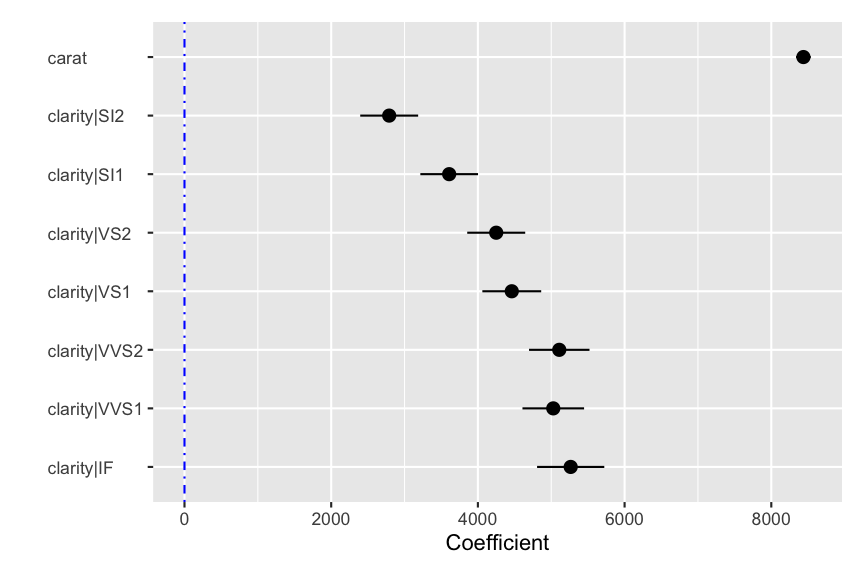``plot(result, plots = "dashboard", lines = "line", nrobs = 100)``